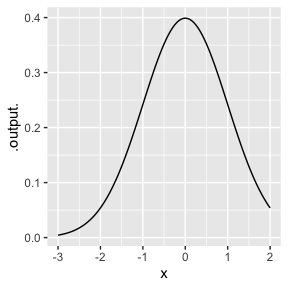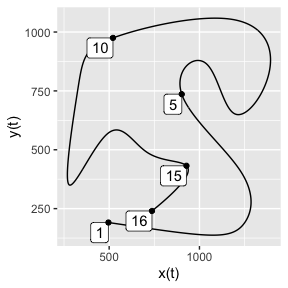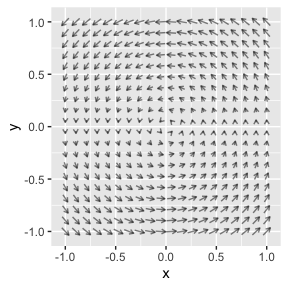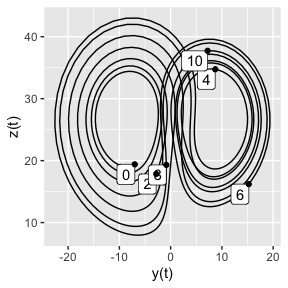# Instructors’ Guide

This guide is intended for instructors who plan to use the {mosaicCalc} package for teaching calculus or related disciplines. The main point of the guide is to make explicit the conventions used in {mosaicCalc}, so that they can be better emphasized to students. If you are an instructor (or student) just getting started with R, better to start with the “Calculus with R” vignette.

## Functions and tilde expressions

There are many ways to define calculus, the perspective taken in {mosaicCalc} and the associated MOSAIC Calculus textbook puts continuous functions at the core. Consequently, the representation of mathematical functions is a central design choice for {mosaicCalc}.

Consider the case of defining R versions of two mathematical functions $f(x) \equiv a x + b\ \ \ \ \text{and}\ \ \ \ g(t) \equiv a e^{-k t}\ .$

A “natural” but misguided way to translate these definition into R is

# misguided!
f0 <- function(x) a*x + b
g0 <- function(t) a*exp(-k*t)

The problem here is that the parameter a will always be numerically the same in both $$f()$$ and $$g()$$. Avoiding such linkage in this style of function definition would require new names for parameters in every new function. That is unsustainable, since constructing a new function would involve knowing all other functions in order to avoid their parameter names.

R offers a native solution to this unintended linkage problem by declaring parameters to be arguments to functions. In this style, the definitions would be

f1 <- function(x, a, b) a*x + b
g1 <- function(t, k, a) a*exp(-k*t)

Even better is when numerical values can be assigned to the parameters when the function is constructed, for instance

f2 <- function(x, a=2, b=-3) a*x + b
g2 <- function(t, k=0.5, a=10) a*exp(-k*t)

This way, functions f2() and g2() can be used with a single argument.

You can successfully use the parameter-as-argument style of function definition with {mosaicCalc}, but the {mosaic} suite of packages offer an alternative:

f3 <- makeFun(a*x + b ~ . , a=2, b=3)
g3 <- makeFun(a*exp(-k*t) ~ . , a=10, k=0.5)

Notice that the formulas inside f3() and g3() are being conveyed to makeFun() as tilde expressions, about which we’ll say more later. (Experienced R programmers will recognize that what we call a “tilde expression” is conventionally called a “formula.” We prefer “tilde expression” because in calculus we need to use the word “formula” in its mathematical, not R-computing, sense.)

In calculus, an everyday task is constructing new functions out of old ones. For instance, we might need to use the function $$f(x) g(t)$$. With a tilde expression, this product of functions can be constructed at a stroke, as in these calculations of the mixed partial derivative:

D(f3(x)*g3(t) ~ x & t)
#> function (x, t)
#> -10 * exp(-0.5 * t)
D(f3(x, a=10) * g3(t, k=1) ~ x & t)
#> function (x, t)
#> -100 * exp(-t)

## Bounds

Many tasks using functions require specifying a region of the function’s domain. For instance, drawing the graph of a function requires specifying a finite region. Similarly, “definite integration”, for example $\int_0^\infty \text{gaussian}(x)\,dx\ ,$ involves the region $$0 \leq x < \infty$$ between the “limits of integration” or “bounds of integration.” In {mosaicCalc}, regions are specified using the R/mosaicCalc function bounds(). The following illustrates simple operations on dnorm(), the R name for the Gaussian function.

slice_plot(dnorm(x) ~ x, bounds(x=-3:2))Integrate(dnorm(x) ~ x, bounds(x=-2:2))
#>  0.9544997

When there are multiple inputs to be bounded, list them all in the same call to bounds(). For instance:

contour_plot(cos(x)*exp(-t) ~ x & t, bounds(x=0:10, t=0:2))

Several things to point out about bounds() and its use …

1. bounds() is always called as a function. It is invalid to use a named-argument syntax like bounds = 0:10.
2. bounds() requires that the quantity being referred to, x and t in the above example, be explicit.
3. When there is more than one quantity being bounded, all must be stated within a single call to bounds().
4. The preferred syntax in bounds() is something like bounds(x=0.5:3.4), using the colon (:) operator. The : operator when used in this context is equivalent to c(0.5, 3.4).
5. Two other valid constructs in arguments to bounds() have the form bounds(x=c(0.5, 3.4)) and bounds(x=2.5 %pm% 1). The latter is helpful when you want to zoom in on a region. The c(0.5, 3.4) is unnecessarily verbose. (It also can lead to mistakes. Try bounds(x=c(5:3.4)) to see what goes amiss.) The special interpretation of the colon notation within bounds() is entirely to facilitate brevity: bounds() gets used a lot!
6. In every {mosaicCalc} function that requires a region be specified, the call to bounds() must come immediately after the tilde expression(s).
7. For backwards compatibility, domain() has been made a synonym for bounds(). domain() was used in a previous development version of mosaicCalc which was never published to CRAN.

## Varied structures of tilde expressions

Many R users encounter statistical functions such as lm() that take as a first argument a tilde expression. For instance, the statement

lm(mpg ~ wt + cyl, data = mtcars)
#>
#> Call:
#> lm(formula = mpg ~ wt + cyl, data = mtcars)
#>
#> Coefficients:
#> (Intercept)           wt          cyl
#>      39.686       -3.191       -1.508

constructs a linear model using mpg as the response variable with wt and cyl as the explanatory variables. In fact, tilde expressions are of very general use, whenever it is desired to construct a symbolic statement, that is a statement that will not be evaluated by the R interpreter but remains as a fragment of code.

{mosaicCalc} makes extensive use of symbolic statements, since they are a close analog to mathematical formulas. This section outlines the idioms of tilde expressions in {mosaicCalc} functions. Knowing the common patterns can help you avoid errors.

The R-language mandates that every tilde expression have a right-hand side that is a syntactically correct expression. For example, ~ 1 and ~ sqrt(foobar) are correct possibilities, but, for example, 1 ~ (without a right-hand side) and ~ 1b are not correct.

The left-hand side of the tilde expression, if present at all, must similarly be syntactically correct.

All tilde expressions used with mosaicCalc functions must be two-sided.1 The two sides stand for different things.

One side of a {mosaicCalc} tilde expression is always the formula for a function (in a mathematical sense). Let’s be clear about what this means. In the mathematical definition $f(x) \equiv mx + b\ ,$ the formula is $$mx + b$$. As an R expression, this translates to m*x + b.

The other side of a tilde expression sets a context. Examples demonstrate the various contexts available.

1. Context: Define a function using makeFun(). The left side of the tilde expression is a formula and the right side should be .. For instance:
f <- makeFun(m*x + b ~ .)
f
#> function (x, b, m)
#> m * x + b
#> <environment: 0x7fb83028ced8>

It’s also possible to have a right-hand side that’s not a period. Whatever names appear in the right-hand side, in the order in which they appear, will become the arguments to the resulting function in that same order. To illustrate,

makeFun(m*x + b ~ b/(m*x)) # bad style!
#> function (b, m, x)
#> m * x + b

More typically, if you want to specify the order of the arguments, you would use a right-hand side like b & m & x.

1. Context: Graph a function with, e.g., slice_plot() or contour_plot(). The left side is a formula and the right side specifies the variable or variables with respect to which the graph is to be made. For instance, in graphing $$mx + b$$ against $$x$$, the appropriate tilde expression is m*x + b ~ x.

2. Context: Differentiation or Integration. These operations always involve a “with respect to variable.” Classically, differentation of $$f()$$ with respect to $$x$$ is written $$df/dx$$ and integration is written $$\int f(x) dx$$. Neither of these notations is naturally suited to the creation of computer commands by keyboard, so {mosaicCalc} provides various operators such as D() and antiD() to carry out the calculation. Both of these accept tilde expressions where the left-hand side is a formula and the right-hand side is the with-respect-to variable. For instance:

# Defining a function
g <- makeFun(1/x ~ .)
# Differentiation
D(g(x) ~ x)
#> function (x)
#> -1/x^2
D(g(x) ~ x & x) # 2nd derivative
#> function (x)
#> 2/x^3
# Integration
antiD(g(x) ~ x)
#> function (x, C = 0)
#> log(x) + C
1. Context: Paths or Parametric plots. The {mosaicCalc} traj_plot() function can plot out a path in $$(x,y)$$ space when the functions $$x(t)$$ and $$y(t)$$ are available. Often, these are called “parametric plots” where, in this example, the parameter is $$t$$. Another term in use is “trajectory.” The left-hand side of the tilde expression is $$y(t)$$, as with slice_plot(). The right-hand side of the tilde expression is $$x(t)$$. Note that the ordinary slice plot is equivalent to the tilde expression y(t) ~ t. (Unlike the slice plot, traj_plot() will mark discrete values of t along the path.)
x <- spliner(x ~ t, data = Robot_stations) # makes a function from data
y <- spliner(y ~ t, data = Robot_stations) # ditto
traj_plot(y(t) ~ x(t), domain(t=1:16))1. Context: Vector fields and ODEs.

A vector field is the assignment of a vector to each point in a region of space. Since {mosaicCalc} graphics are two-dimensional, specifying a vector field is a matter of defining functions giving the two components of the vectors. This is done by specifying two tilde expressions, one for each component of the vector. Like this:

vectorfield_plot(x ~ -y, dy ~ x, domain(x=-1:1, y=-1:1))The first tilde expression specifies the first component of the vector, the second tilde expression gives the second component. The function itself is on the left-hand side of the tilde expression. The right side names the two inputs to those functions, as with contour_plot().

1. Context: Differential equations. The workhorse function for solving ordinary differential equations in {mosaicCalc} is integrateODE(). ODEs can involve multiple dynamical variables. For integrateODE(), each dynamical variable must have its own tilde expression. These tilde expression have a distinctive format.

The left-hand side will be the letter “d” preceeding the name of a dynamical variable. The right-hand side will be the function of the dynamical variables that gives the time derivative of the variable indicated by the left-hand side. There must be as many tilde expressions as there are dynamical variables, one tilde expression for each variable. For example, here are the famous Lorenz equations from chaos theory, with state variables $$x$$, $$y$$, and $$z$$.

T1 <- integrateODE(dx ~ sigma*(y-x),    # x dynamics
dy ~(x*(rho-z) - y), # y dynamics
dz ~ (x*y - beta*z), # z dynamics
domain(t=0:10),
x=-5, y=-7, z=19.4,  # initial conditions
rho=28, sigma=10, beta = 8/3) # parameters
#> Solution containing functions x(t), y(t), z(t).
traj_plot(z(t) ~ y(t), T1, npts=1000)   # T1 is the solution## Parameters and binding

Consider a function that might be written conventionally as $f(x) \equiv a x^2 + b x + c\ .$ According to the convention, $$x$$ is the input and $$a, b, c$$ are parameters. Many instructors will want to teach this convention to their students. This section is not about the virtues or demerits of the convention, but only about the implementation in {mosaicCalc}.

The {mosaicCalc} principle is very simple: there is no operational distinction between inputs and parameters. This principle is not a statement about mathematical pedagogy. Instead, it reflects the desire to avoid having to teach the concept of “scope” in computing languages.

Implementing in R the above function $$f(x)$$ in a natural way is accomplished with

f <- makeFun(a*x^2 + b*x + c ~ .)
f
#> function (x, a, b, c)
#> a * x^2 + b * x + c
#> <environment: 0x7fb827d15a30>

Notice that f() has four arguments. What we conventionally call “parameters” are implemented as arguments.

Often, parameters represent some physical constant. When this is the case, the user has a choice of writing the constant explicitly or representing it with a symbol. For instance, a function giving the y-position of a falling object might be written like this:

y <- makeFun(-9.8*t^2/2 + v0*t + y0 ~ .)
y
#> function (t, v0, y0)
#> -9.8 * t^2/2 + v0 * t + y0
#> <environment: 0x7fb827f244b0>

or like this:

y <- makeFun(g*t^2/2 + v0*t + y0 ~ ., g=-9.8)
y
#> function (t, g = -9.8, v0, y0)
#> g * t^2/2 + v0 * t + y0
#> <environment: 0x7fb826acc0c0>

The quantity 9.8 (m/s2) is the “acceleration due to gravity” near the surface of the Earth. This is typically named $$g$$. Physically, $$v_0$$ and $$y_0$$ stand for the initial vertical velocity and position respectively. In the second definition of y(), the value of $$g$$ is specified outside of the tilde expression.

We call parameters like $$g$$ bound parameters when a numerical value has been assigned to them outside the tilde expression. In contrast, unbound parameters are those to which no numerical value has been assigned. $$v_0$$ and $$x_0$$ are examples of unbound parameters.

As the y() examples show, you do not need to bind all parameters at the time you define a function. Similarly, you do not need to bind all parameters to undertake a symbolic calculation. For example, here is the velocity function $$v_y(t)$$ as derived from $$y(t)$$:

v_y <- D(y(t) ~ t)
v_y
#> function (t, g = -9.8, v0, y0)
#> g * t + v0

For numerical operations, however, all parameters must be bound. Typically, {mosaicCalc} operations allow you to bind (or re-bind) parameters at any stage, and they keep track of the most recent bindings. For instance, to find a zero crossing for $$v_y(t)$$, we need to bind a numerical value to the parameter v0. Suppose that is to be $$v_0 = 20$$ m/s2. The calculation requires that a value be given to $$v_0$$, like this:

Zeros(v_y(t, v0=20) ~ t, domain(t=0:20))

Or, if we wanted to know the situation on the moon, where the acceleration due to gravity is -1.6 m/s2, we write

Zeros(v_y(t, v0=20, g=-1.6) ~ t, bounds(t=0:20))
#> # A tibble: 1 × 2
#>       t .output.
#>   <dbl>    <dbl>
#> 1  12.5        0

Notice that the value of $$g$$ is specified in the arguments to $$v_y()$$. It will not work to bind the parameter $$g$$ to $$v_y()$$ in the numerical function. For instance, this generates an error:

Zeros(v_y(t, v0=20, g=-1.6) ~ t, bounds(t=0:20))
#> # A tibble: 1 × 2
#>       t .output.
#>   <dbl>    <dbl>
#> 1  12.5        0

A working alternative is to build the velocity function with the new, moon binding built in. Like this:

v_y_on_moon <- D(y(t) ~ t, g=-1.6)
v_y_on_moon
#> function (t, g = -1.6, v0, y0)
#> g * t + v0

Then, with a default moon value for $$g$$ in v_y_on_moon(), we need only specify the initial velocity to find the zero crossing:

Zeros(v_y_on_moon(t, v0=20) ~ t, bounds(t=0:20))
#> # A tibble: 1 × 2
#>       t .output.
#>   <dbl>    <dbl>
#> 1  12.5        0

It is admittedly confusing that you can’t bind arguments at the phase where a numerical evaluation is being done. The reason for this is technical, viz, a function $$g()$$ with bound parameters might be used in the construction of another function $$f()$$ without the parameter being explicitly mentioned in $$f()$$. Therefore, $$f()$$ will not “know” that the bound parameters even exist.

The overall rule is that operations like D(), antiD(), and makeFun(), which build new functions can use the binding syntax (e.g. D(y(t) ~ t, g=-1.6)). Operations like Zeros() or argM() or slice_plot() which don’t build functions cannot use the binding syntax. Instead, they have to set the parameter as an argument to the function in the tilde expression (e.g., slice_plot(v_y(t, v0=20, g=-1.6) ~ t, bounds(t=0:20))).

## Argument order

Students working with {mosaicCalc} will be building mathematical functions and using the functions they build. Since many students will have had no previous experience in defining functions, it is to be expected that they will not start with programming skills such as paying attention to the order of arguments to functions. Getting arguments in the wrong order can lead to frustration and loss of motivation.

Based on experience using {mosaicCalc} to teach calculus to hundreds of students, we think it best to simplify student tasks by automating the process of assigning order to arguments when defining functions. The automation gives preference to names with the traditional single letter: $$x, y, z, t, u, v, w$$ in that order. Any other argument names are sorted alphabetically after the single-letter special cases.

A good practice, when there are multiple arguments to a function, is to evaluate the function using R’s named-argument syntax. For example, accel(vel=2, pos=3) will work whatever the order of the arguments in the definition of ballistics.

1. The only exception is makeFun(), which can optionally be used with one-sided expressions: e.g. makeFun(~ m*x + b). Even here, you can use a two-sided expression, for instance, makeFun(m*x + b ~ .). The two forms are completely equivalent. Note that the period . is a legitimate R expression and thus fulfills the requirement for a two-sided tilde expression.↩︎